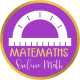Cuboid

Cuboid

A rectangular solid is also called a rectangular prism or cuboid.

In a rectangular solid, the lenght, width and height might be of different lenghts

Volume

The volume is found using the formula:

Inverse formulas:

Surface area

The total surface area (TSA) of a cuboid is the sum of the areas of its six faces.
That is:

For  example:

Find the total surface area of a cuboid with dimensions 8 cm by 6 cm by 5 cm.
TSA = 2 (lw+wh+hl)
TSA = 2 (8⋅6+6⋅5+5⋅8) = 2(48+30+40) = 236 cm2
So, the total surface area of this cuboid is 236 cm2

For  example:

We want to find the volume of this cuboid in cm3

To find the volume we mulptiply the sides.

V=3∙4∙7 = 84 cm3

Exercises

1. A cuboid has lateral surface 199 cm . The base has sides 23 cm and 14 cm long. Calculate the height. Solution
2. A cuboid has its height equal to the diagonal of the base. The sum of the two sides of the base is 21 cm and one is 3/4 of the other. Calculate the total surface area.
3. The diagonal of a cuboid is 117 cm the base has sides 36 cm and 27 cm long. Calculate the volume and the total surface area.
4. A cuboid has the perimeter of the base equal to 84 dm and one side is 3/4 of the other. The height is 40 dm long. Calculate the total surface area.
5. The sum of two sides of a cuboid is 14 cm and one side is 4/3 of the other. Knowing that the total surface area is 768 cm, calculate the third dimension of the cuboid.
6. The difference between two sides of a cuboid is 14 cm and one side 15/8 of the other. The third side (height) is 33 cm long. A second cuboid has a squared base with 121 cmarea and has the same lateral area of the first one. Calculate the third side.
7. A cuboid has two sides 13 cm and 15 cm long. The total surface area is 950 cm. Calculate the volume. Solution
8. The squared base of a cuboid is 576 cm. The height of the cuboid is 1/12 of the perimeter of the base. Calculate lateral surface and volume.
9. The volume of a cuboid is 108 cm3 and two sides are 4.5 cm and 6 cm long. Calculate the diagonal and the total surface area of the cuboid.
10. The volume of a cuboid is 1768 cm3 . The sum and the difference between two sides are 30 cm and 4 cm. Calculate the lenght of the third side.
11. The perimeter of the base of a cuboid is 66 cm and one side is 6/5 of the other. The volume is 2.97dm3 . Calculate the lenght of the third side.

2 thoughts on “Cuboid”

1.Sems says:

its fun doing when i have time…

Like

1.matemaths says:

Thank you! I’m trying to be helpful to teacher and students

Like

This site uses Akismet to reduce spam. Learn how your comment data is processed.